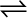7.12 A mixture of 1.57 mol of N2, 1.92 mol of H2 and 8.13 mol of NH3 is introduced into a 20 L reaction vessel at 500 K. At this temperature, the equilibrium constant, Kc for the reaction N2 (g) + 3H2 (g)2NH3 (g) is 1.7 × 102. Is the reaction mixture at equilibrium? If not, what is the direction of the net reaction?

The given reaction is:

${\mathrm{N}}_{2\left(\mathrm{g}\right)}+3{\mathrm{H}}_{2\left(\mathrm{g}\right)}↔2{\mathrm{NH}}_{3\left(\mathrm{g}\right)}$

The given concentration of various species is

Now, the reaction quotient ${Q}_{c}$ is:

Since, ${Q}_{c}\ne {K}_{c}$, the reaction mixture is not at equilibrium.

Again, ${Q}_{c}>{K}_{c}$, Hence, the reaction will proceed in the reverse direction.